# diffusion/laplace_fluid_2d.py¶

Description

A Laplace equation that models the flow of “dry water” around an obstacle shaped like a Citroen CX.

## Description¶

As discussed e.g. in the Feynman lectures Section 12-5 of Volume 2 (https://www.feynmanlectures.caltech.edu/II_12.html#Ch12-S5), the flow of an irrotational and incompressible fluid can be modelled with a potential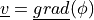that obeys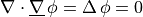The weak formulation for this problem is to find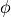such that: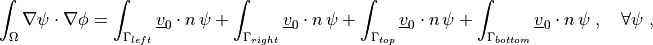where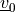is the 2D vector defining the far field velocity that generates the incompressible flow.

Since the value of the potential is defined up to a constant value, a Dirichlet boundary condition is set at a single vertex to avoid having a singular matrix.

## Usage examples¶

This example can be run with the following:

sfepy-run sfepy/examples/diffusion/laplace_fluid_2d.py
sfepy-view citroen.vtk -f phi:p0 phi:t50:p0 --2d-view


## Generating the mesh¶

The mesh can be generated with:

gmsh -2 -f msh22 meshes/2d/citroen.geo -o meshes/2d/citroen.msh
sfepy-convert --2d meshes/2d/citroen.msh meshes/2d/citroen.h5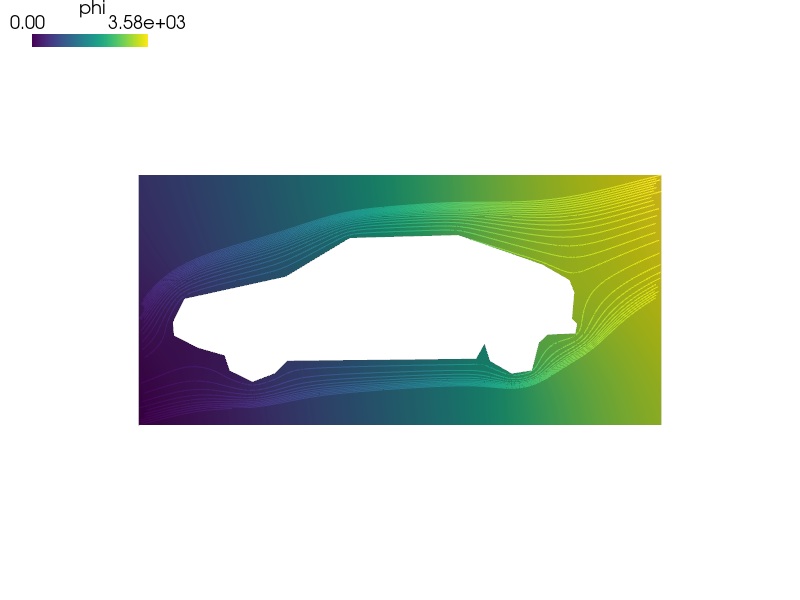source code

r"""
A Laplace equation that models the flow of "dry water" around an obstacle
shaped like a Citroen CX.

Description
-----------

As discussed e.g. in the Feynman lectures Section 12-5 of Volume 2
(https://www.feynmanlectures.caltech.edu/II_12.html#Ch12-S5),
the flow of an irrotational and incompressible fluid can be modelled with a
potential :math:\ul{v} = \ul{grad}(\phi) that obeys

.. math::
\nabla \cdot \ul{\nabla}\,\phi = \Delta\,\phi = 0

The weak formulation for this problem is to find :math:\phi such that:

.. math::
\int_{\Omega} \nabla \psi \cdot \nabla \phi
= \int_{\Gamma_{left}} \ul{v}_0 \cdot n  \, \psi
+ \int_{\Gamma_{right}} \ul{v}_0 \cdot n  \, \psi
+ \int_{\Gamma_{top}} \ul{v}_0 \cdot n \,\psi
+ \int_{\Gamma_{bottom}} \ul{v}_0 \cdot n \, \psi

where :math:\ul{v}_0 is the 2D vector defining the far field velocity that
generates the incompressible flow.

Since the value of the potential is defined up to a constant value, a Dirichlet
boundary condition is set at a single vertex to avoid having a singular matrix.

Usage examples
--------------

This example can be run with the following::

sfepy-run sfepy/examples/diffusion/laplace_fluid_2d.py
sfepy-view citroen.vtk -f phi:p0 phi:t50:p0 --2d-view

Generating the mesh
-------------------

The mesh can be generated with::

gmsh -2 -f msh22 meshes/2d/citroen.geo -o meshes/2d/citroen.msh
sfepy-convert --2d meshes/2d/citroen.msh meshes/2d/citroen.h5
"""
import numpy as nm
from sfepy import data_dir

filename_mesh = data_dir + '/meshes/2d/citroen.h5'

v0 = nm.array([1, 0.25])

materials = {
'm': ({'v0': v0.reshape(-1, 1)},),
}

regions = {
'Omega': 'all',
'Gamma_Left': ('vertices in (x < 0.1)', 'facet'),
'Gamma_Right': ('vertices in (x > 1919.9)', 'facet'),
'Gamma_Top': ('vertices in (y > 917.9)', 'facet'),
'Gamma_Bottom': ('vertices in (y < 0.1)', 'facet'),
'Vertex': ('vertex in r.Gamma_Left', 'vertex'),
}

fields = {
'u': ('real', 1, 'Omega', 1),
}

variables = {
'phi': ('unknown field', 'u', 0),
'psi': ('test field', 'u', 'phi'),
}

# these EBCS prevent the matrix from being singular, see description
ebcs = {
'fix': ('Vertex', {'phi.0': 0.0}),
}

integrals = {
'i': 2,
}

equations = {
'Laplace equation':
"""dw_laplace.i.Omega( psi, phi )
= dw_surface_ndot.i.Gamma_Left( m.v0, psi )
+ dw_surface_ndot.i.Gamma_Right( m.v0, psi )
+ dw_surface_ndot.i.Gamma_Top( m.v0, psi )
+ dw_surface_ndot.i.Gamma_Bottom( m.v0, psi )"""
}

solvers = {
'ls': ('ls.scipy_direct', {}),
'newton': ('nls.newton', {
'i_max': 1,
'eps_a': 1e-10,
}),
}